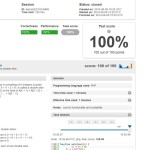# MaxSliceSum Demo Task at Codility.comCodility.com Tests 100/100 Solutions

Lesson 7 – Maximum slice problem – MaxSliceSum demo task solution code written in php. Gives 100/100 score at the time of publishing.

Solution in php:

```function solution(\$A) {
\$cnt = count(\$A);
\$max_ending_here = \$A;
\$max_so_far = \$A;
for(\$i = 1; \$i < \$cnt; \$i++){
\$max_ending_here = max(\$A[\$i], \$max_ending_here + \$A[\$i]);
\$max_so_far = max(\$max_so_far, \$max_ending_here);
}
return \$max_so_far;
}
```

Given “AS IS”, can be ported from other languages from solutions found on the internet, please use with care.

Please note: we think that codility.com does not give a correct assessment of your real-world programming skills. For instance, reading the below would bring more understanding why: //x20x.co.uk/2014/02/why-i-refuse-to-use-codility-and-so-should-you/